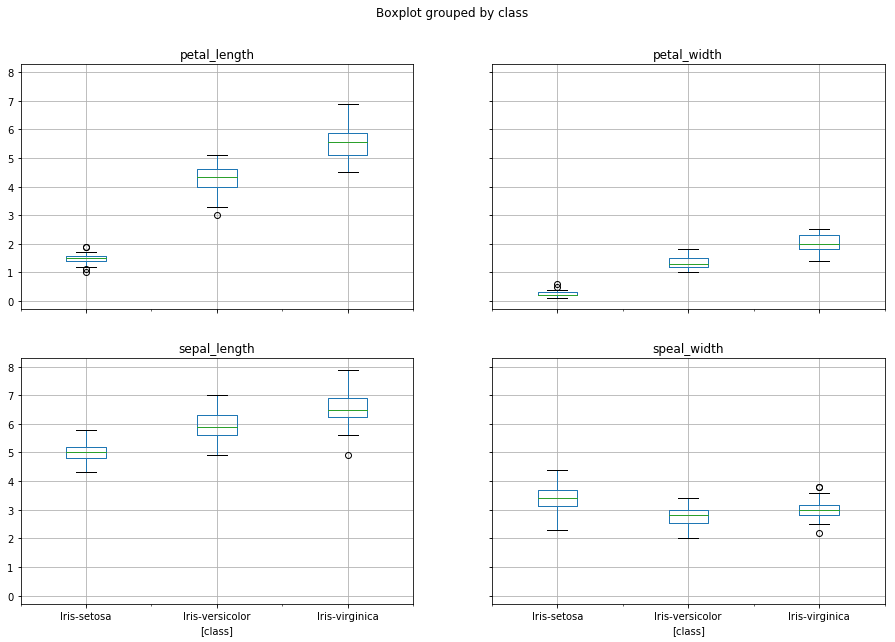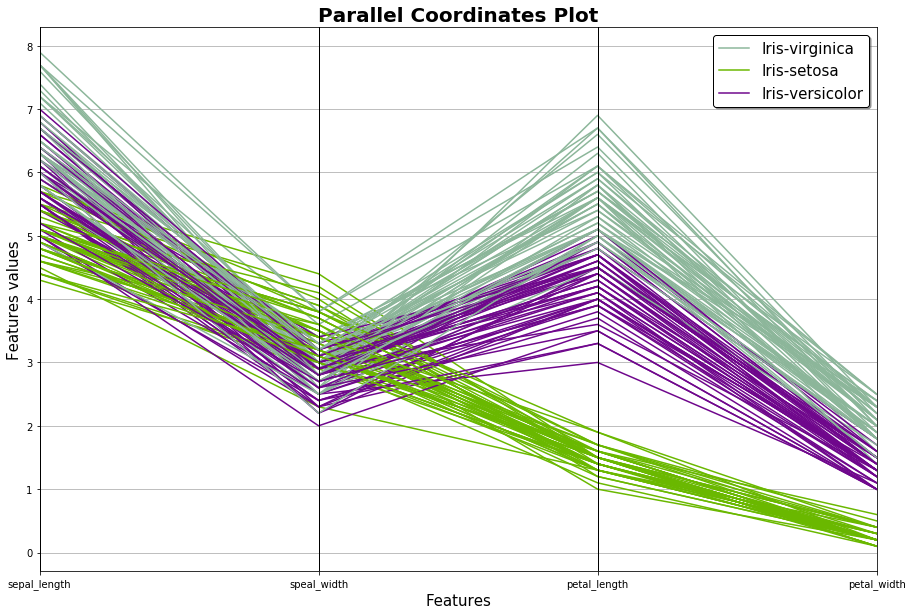# K-Nearest Neighbors, Boxplot, Standard Score, Feature Scaling, Curse of Dimensionality, Missing Values, Confusion Matrix, Classification Report, ROC-Curve, AUROC¶

Today's data is one of the most used data sets in Machine Learning examples about classification. It's about beautiful Iris flowers and how they can be classified into different types of Iris flowers. This is the url where the data and the attribute information can be found: https://archive.ics.uci.edu/ml/machine-learning-databases/iris/ Nevertheless, you can also simply continue with this tutorial because all necessary information are also provided here.

### Attribute information¶

1. sepal length in cm
2. sepal width in cm
3. petal length in cm
4. petal width in cm
5. class: -- Iris Setosa -- Iris Versicolour -- Iris Virginica

In :
import pandas as pd

#attribute names
names = ['sepal_length', 'speal_width', 'petal_length', 'petal_width', 'class']

#get data with attribute names
iris_data = pd.read_csv('iris_data.csv', names = names)

#shuffle data
iris_data = iris_data.sample(frac=1)

#print 5 first lines of the data

Out:
sepal_length speal_width petal_length petal_width class
138 6.0 3.0 4.8 1.8 Iris-virginica
2 4.7 3.2 1.3 0.2 Iris-setosa
94 5.6 2.7 4.2 1.3 Iris-versicolor
107 7.3 2.9 6.3 1.8 Iris-virginica
125 7.2 3.2 6.0 1.8 Iris-virginica

### Divide into features and target¶

In :
#exctract feature variables
x_variables = iris_data.loc[:, iris_data.columns != 'class']

#extract target variable
y_variable = iris_data['class']


### Split into training and test data¶

In :
from sklearn.model_selection import train_test_split

#get training and test data
x_train, x_test, y_train, y_test = train_test_split(x_variables, y_variable, test_size=0.20)


## Standard score (z-score) and Feature Scaling¶

Great! We already have our data, we split it into feature and target variables as well as into test and training data. Before we learn how the K-Nearest Neighbors algorithm works, we will have a look at Normalization, what it is and why it is done. Imagine you have features that vary in magnitudes, units and range. Classification calculations could become difficult, for example, if you want to classify a person using weight and height into overweight, normal weight and underweight: centimetres and kilograms are different units and the amount of kilograms that a person weighs is usually less than half of the number of centimetres of a person's height. If we want to make the numbers of two features more comparable we somehow have to scale them to the same level. The most popular ways to do this are Standardization and Feature Scaling: The formula for the Standard Score looks like this:
$z={x-\mu \over \sigma }$ where $\mu$ is the population mean and $\sigma$ is the population standard deviation. After applying this formula the mean becomes 0 and the standard deviation 1. The cool thing about the z-score is that it made the data become much more comparable. For example, if you know that someone's weight z-score is 3, then you know that it is 3 standard deviations above the mean. This is quite a lot! If the distribution is somewhat similar to the Gaussian Normal Distribution then the person's weight being 3 standard deviations away from the mean would tell us that that person's weight is higher than the weight of around 98% of the overall population. Using the z-score has the advantage that outliers have less effect when normalizing data than they have when using Feature Scaling for normalization because it has a wider range of possible values. Nevertheless, this wider range might also give more weight to features with a less equal distribution around the mean due to the higher standard deviations. Thus, especially when applying an algorithm that computes distances Feature Scaling probably is the better choice: The formula for Feature Scaling looks like this:
$X'={\frac {X-X_{\min }}{X_{\max }-X_{\min }}}$ After applying this formula all feature values will be in the range [0, 1] or [−1, 1]. Since the K-Nearest Neighbors algorithm computes distances, we will use Feature Scaling. Let's find out how a Python implementation of feature scaling using the Sklearn library looks like.

In :
from sklearn.preprocessing import MinMaxScaler

#create MinMaxScaler object
scaler_min_max = MinMaxScaler()

#fit object to data
scaler_min_max.fit(x_train)

#get transformed train data
x_train_normalized = scaler_min_max.transform(x_train)

#get transformed test data
x_test_normalized = scaler_min_max.transform(x_test)


## K-Nearest Neighbors¶

Now that we have normalized data we can start building our model. Well, actually using the term "building a model" is not really accurate when applying the K-Nearest Neighbors algorithm. The information about the training data is simply stored somewhere - e.g. in a database. Whenever we want to predict the class membership of a new instance we compare the instance with the stored data of the other instances. The most similar instances and their class membership then decide about the new instance's class membership. How is this similarity being measured? Do you remember the Pythagorean theorem? Exactly: $a^2+b^2 = c^2$! This works perfectly for two dimensions. Applying this to a coordinate system in which we want to measure the distance between two points which represent two instances A(2,3) and B(1,2) the distance would be $\sqrt{(2-1)^2 + (3-2)^2}$. By adding one more dimension which is equal to adding a third feature our points (instances) in the coordinate system could for example look like this: A(2,3,5) and B(1,2,7). The new distance would then be $\sqrt{(2-1)^2 + (3-2)^2 +(5-7)^2}$. The idea is the same as with the Pythagorean theorem - only the name for the formula became another one: the Euclidean Distance. Thus, now we also know why it was so important to normalize the data before applying the algorithm: If one feature has values between 1000 and 2000 and another feature values between 0 to 5, then the feature with the values ranging from 0 to 5 would not be significant anymore when calculating the differences of the distances to the new instance. Therefore, in order to maintain the importance of each feature, their range is equally being normalized to values between 0 and 1. Now that we know about all of this we can finally build our KNeighborsClassifier object using the Sklearn library. As a parameter we can decide how many nearest neighbors and their class memberships will be taken into account when deciding on the new instance's class memebership. How many neighbors should be taken into account depends largely on the data - there is no universal rule - it is all about trying. By specifying a "weights" parameter it is also possible to specify that the nearest neighbors that are closer than the other nearest neighbors get more weight in determining the class membership.

In :
from sklearn.neighbors import KNeighborsClassifier

#create KNeighborsClassifier object
classifier_normalization = KNeighborsClassifier(n_neighbors=10)

#fit object to data
classifier_normalization.fit(x_train_normalized, y_train)

Out:
KNeighborsClassifier(algorithm='auto', leaf_size=30, metric='minkowski',
metric_params=None, n_jobs=None, n_neighbors=10, p=2,
weights='uniform')
In :
#get predicitons
y_pred_normalization = classifier_normalization.predict(x_test_normalized)

In :
from sklearn.metrics import classification_report, confusion_matrix

#confusion matrix
print(confusion_matrix(y_test, y_pred_normalization))

#classifiaction report
print(classification_report(y_test, y_pred_normalization))

[[ 9  0  0]
[ 0  8  0]
[ 0  1 12]]
precision    recall  f1-score   support

Iris-setosa       1.00      1.00      1.00         9
Iris-versicolor       0.89      1.00      0.94         8
Iris-virginica       1.00      0.92      0.96        13

micro avg       0.97      0.97      0.97        30
macro avg       0.96      0.97      0.97        30
weighted avg       0.97      0.97      0.97        30



Ok, so far the only thing we know is that apparently the k-nearest neighbors algorithm works really well on our data but some visualization to understand better why this is the case might also be interesting. So let's do it. Before we start there is to mention that this part of the tutorial got most of its inspiration from this website: https://www.kaggle.com/skalskip/iris-data-visualization-and-knn-classification

We start with simple boxplots that show how the range of each feature's charactersitic varies in each class memebership.

In :
import matplotlib.pyplot as plt

#make 4 different boxplots grouped by class memebership
plt.figure()
iris_data.boxplot(by="class", figsize=(15,10))
plt.show()

<Figure size 432x288 with 0 Axes>This looked rather boring so let's do something a little bit fancier. The description of what you can see is cited from this page: (https://www.kaggle.com/skalskip/iris-data-visualization-and-knn-classification, 31.01.2019). "Parallel coordinates is a plotting technique for plotting multivariate data. It allows one to see clusters in data and to estimate other statistics visually. Using parallel coordinates points are represented as connected line segments. Each vertical line represents one attribute. One set of connected line segments represents one data point. Points that tend to cluster will appear closer together."

In :
from pandas.plotting import parallel_coordinates

#figure size
plt.figure(figsize=(15,10))

#define features and class
parallel_coordinates(iris_data, "class")

#Plot title
plt.title('Parallel Coordinates Plot', fontsize=20, fontweight='bold')

#name x-axis
plt.xlabel('Features', fontsize=15)

#name y-axis
plt.ylabel('Features values', fontsize=15)

#legend attributes definiton
plt.legend(loc=1, prop={'size': 15}, frameon=True,shadow=True, facecolor="white", edgecolor="black")
plt.show()Ok, this already looked much fancier! But we are still caught in a 2D world! Let's add a third dimension! Well, actually we have 4 features. Thus, a fourth dimension would be even better. Unfortunately no human being - at least as far as I know - is capable of imagining something like this. Thus, a little trick was used in the 3D plot: Reagrding the fourth feature its values are being representated by the size of the data points.

Most of the time it is much easier for machine learning models to handle numeric data rather than string data. In this case our 3D plot can not handle string data in order to give different colors to the data points. That's why we use the LabelEncoder object to transform the class membership names into numbers from 0-2.

## Curse of Dimensionality¶

Looking at the 3D plot we can see how small the number of instances appears to be in the big space which the 3D plot provides. Imagine how little the amount of data would look like if we added even more dimensions. Well, yes, you are right, you cannot imagine how that might look like because we are unable to imagine a space with more than three dimensions. Nevertheless, I hope this explained the point: The more dimensions you create by adding more features the more instances you need to fill the space and therefore to be able to make valuable class distinctions. Therefore, when applying the k-nearest neighbor algorithm, it can be very useful to preselect relevant features wisely instead of using all features you have. Remember the earlier example about classifying people into underweight, normal weight and overweight: height and weight definitely seem like important features. However, if we also had information about the person's favourite animal, this might not be relevant for our classification and this feature could be left out for better results.

In :
from sklearn.preprocessing import LabelEncoder

le = LabelEncoder()
y = le.fit_transform(y_variable)

#find out which order classes have
print(le.classes_)

from mpl_toolkits.mplot3d import Axes3D
fig = plt.figure(1, figsize=(20, 15))
ax = Axes3D(fig, elev=48, azim=134)
ax.scatter(x_variables.iloc[:,0], x_variables.iloc[:,1], x_variables.iloc[:,2], c = y,
cmap=plt.cm.Set1, edgecolor='k', s = x_variables.iloc[:, 3]*150)

#get position for class names in plot
for name, label in [('Iris-setosa', 0), ('Iris-versicolor', 1), ('Iris-virginica', 2)]:
ax.text3D(x_variables.iloc[y == label, 0].mean(),
x_variables.iloc[y == label, 1].mean(),
x_variables.iloc[y == label, 2].mean(), name,
horizontalalignment='center',
bbox=dict(alpha=.5, edgecolor='w', facecolor='w'),size=25)

ax.set_title("3D visualization", fontsize=40)
ax.set_xlabel("Sepal Length [cm]", fontsize=25)
ax.w_xaxis.set_ticklabels([])
ax.set_ylabel("Sepal Width [cm]", fontsize=25)
ax.w_yaxis.set_ticklabels([])
ax.set_zlabel("Petal Length [cm]", fontsize=25)
ax.w_zaxis.set_ticklabels([])

plt.show()

['Iris-setosa' 'Iris-versicolor' 'Iris-virginica']